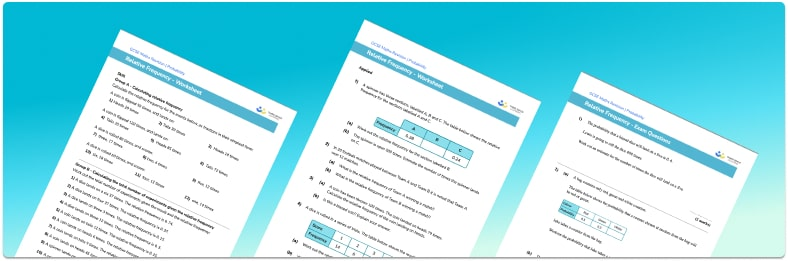# Relative Frequency Worksheet• Section 1 of the relative frequency worksheet contains 20+ skills-based relative frequency questions, in 3 groups to support differentiation
• Section 2 contains 3 applied relative frequency questions with a mix of worded problems and deeper problem solving questions
• Section 3 contains 4 foundation and higher level GCSE exam style relative frequency questions
• Answers and a mark scheme for all relative frequency questions are provided
• Questions follow variation theory with plenty of opportunities for students to work independently at their own level
• All questions created by fully qualified expert secondary maths teachers
• Suitable for GCSE maths revision for AQA, OCR and Edexcel exam boards

• This field is for validation purposes and should be left unchanged.

You can unsubscribe at any time (each email we send will contain an easy way to unsubscribe). To find out more about how we use your data, see our privacy policy.

### Relative frequency at a glance

Relative frequency, also called experimental probability, is the probability of an event occurring based on previous events. This is useful when we don’t know the theoretical probability of an event occurring. For example, let’s say we have a biased spinner with three possible outcomes: red, green, and blue. We do not know the probability of each outcome but when we spin the spinner 100 times it lands on red 38 times. Then we can say the relative frequency of landing on red is 38 out of 100.

Information about the frequency of events and relative frequencies can be recorded in a relative frequency table.

We can use information from a relative frequency table to suggest whether or not something is biased. The key is to look out for the probability of a random variable that is significantly different from the rest of the probabilities or knowing what the expected probability of the event should be compared to the experimental probability. For example, if we rolled a dice 600 times and a 4 was rolled 300 times, the experimental probability of rolling a 4 would be a half (300 out of 600). If this was a fair die, the number of times a 4 should be rolled should be very close to, if not exactly, 100 as this is equivalent to one sixth of the total number of rolls.

As the total number of trials increases, the accuracy of the theoretical probability improves.

Looking forward, students can then progress to additional probability worksheets, for example the mutually exclusive events worksheet, the and / or rule probability worksheet, or the experimental probability worksheet.For more teaching and learning support on probability our GCSE maths lessons provide step by step support for all GCSE maths concepts.

## Do you have KS4 students who need more focused attention to succeed at GCSE?There will be students in your class who require individual attention to help them succeed in their maths GCSEs. In a class of 30, it’s not always easy to provide.

Help your students feel confident with exam-style questions and the strategies they’ll need to answer them correctly with our dedicated GCSE maths revision programme.

Lessons are selected to provide support where each student needs it most, and specially-trained GCSE maths tutors adapt the pitch and pace of each lesson. This ensures a personalised revision programme that raises grades and boosts confidence.

Find out more